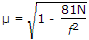# Electronics and Communication Engineering - Electromagnetic Field Theory

1.
Distilled water at 25°C is characterized by σ = 1.7 x 10-4 mho/m and ε = 78 ε0 at a frequency of 3 GHz. Its loss tangent is (ε = 10-9/36p F/m)
1.3 x 10-5
1.3 x 10-3
1.7 x 10-4/78
1.7 x l0-4/78ε0
Explanation:

Loss tangent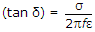.

2.
The input impedance of short-circuited line of length l where l < λ/4
inductive
capacitive
resistive
none of the above
Explanation:

l < λ/4, ZL = 0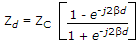, if Zd comes +j kZC then inductive,

If Zd comes -j kZC then capacitive.

For 0 ≤ l ≤ 1/4, λ ≤ l ≤ 3λ/4 will be inductive.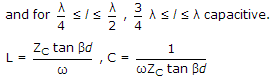3.
If the height of transmitting and receiving antenna in a LOS system are 49 meter and a 9 meter respectively then the distance up to which communication may be possible is about
40 km
110 km
400 km
1100 km
Explanation:

Distance = 0.4 [ht + hr] metre.

4.
In a line VSWR of a load is 6 dB. The reflection coefficient will be
0.033
0.33
0.66
3.3
Explanation:

VSWR = 100.6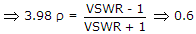5.
The refractive index of E layer at frequency of 81 N
0
1
0.5